# Triangle

Prove whether you can construct a triangle ABC, if a=9 cm, b=10 cm, c=4 cm.

Result

#### Solution:Leave us a comment of example and its solution (i.e. if it is still somewhat unclear...):

Showing 0 comments:Be the first to comment!#### To solve this example are needed these knowledge from mathematics:

See also our trigonometric triangle calculator.

## Next similar examples:

1. Triangle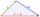Determine if it is possible to construct a triangle with sides 28 31 34 by calculation.
2. Center traverseIt is true that the middle traverse bisects the triangle?
3. Angle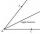Draw angle |∠ ABC| = 130° and built its axis. What angle is between axis angle and arm of angle?
4. Adding shapes5 triangles + 1 square = how many sides in all
5. Triangle ABCIn a triangle ABC side b measure 10 cm less than the side a and side b is half of the side c. Calculate the length of sides if the circumference of the triangle is 42 cm.
6. MedianThe median of the triangle LMN is away from vertex N 84 cm. Calculate the length of the median, which start at N.
7. Garden plotCalculate how many meters of fence need to fence the square garden with length and width of 22 meters.
8. Roman numeralsWrite numbers written in Roman numerals as decimal.
9. Roman numerals 2+Add up the number writtens in Roman numerals. Write the results as a roman numbers.
10. Train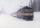The train passes part of the line for 95 minutes at speed 75 km/h. What speed would have to go in order to shorten the driving time of 20 minutes?
11. DoctorsIn the city operates 171 doctors. The city has 128934 citizens. How many citizens are per one doctor?
12. ProductResult of the product of the numbers 1, 2, 3, 1, 2, 0 is:
13. Addition of Roman numbersAdded together and write as decimal number: LXVII + MLXIV
14. To thousands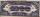Round to thousands following numbers:
15. Compare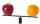Compare with characters >, <, =: 85.57 ? 80.83
16. Roman numerals +Add up the number writtens in Roman numerals. Write the results as a decimal number.
17. Line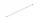How many parts of line divide 5 (different) points that lie on it?